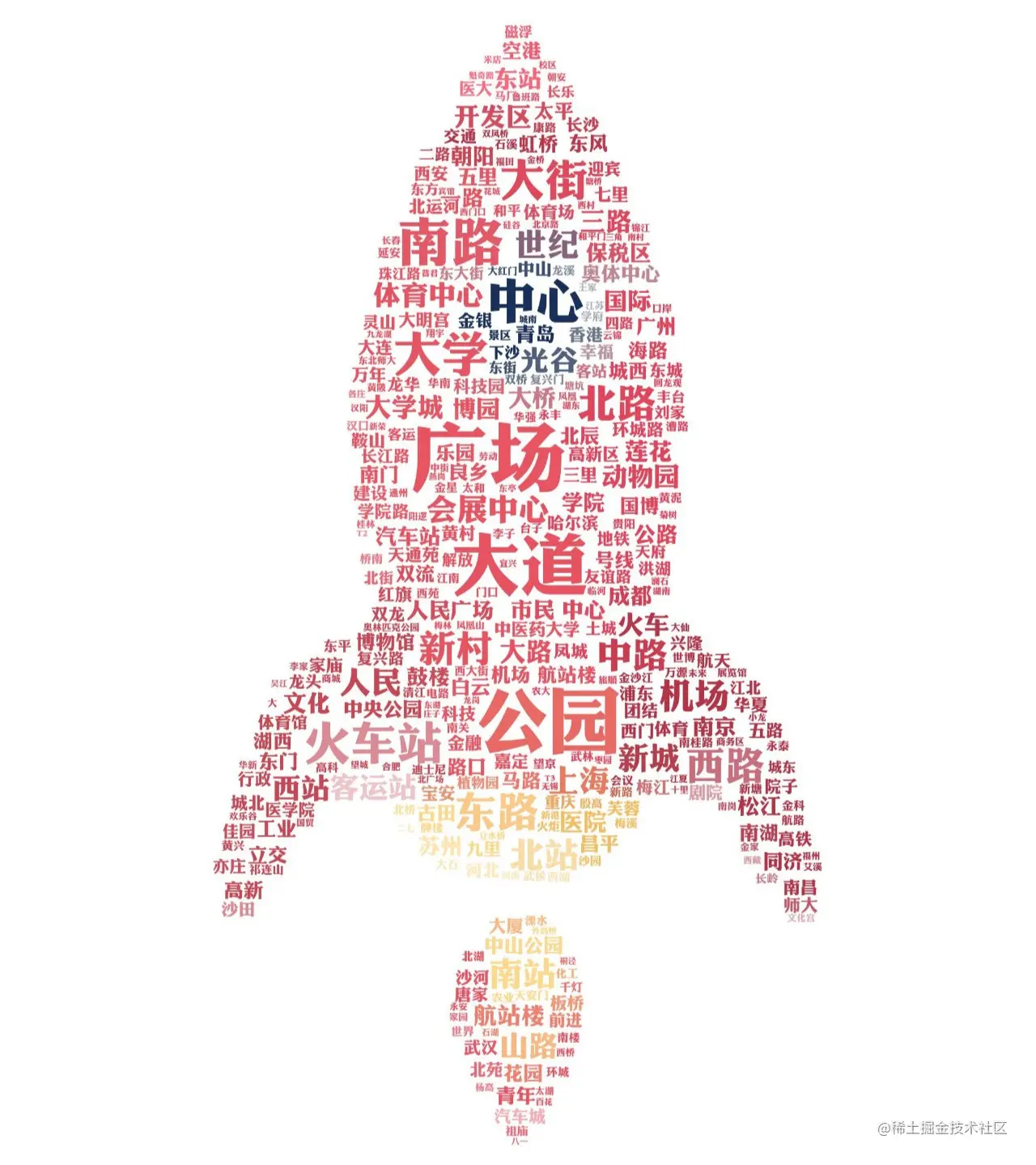# Python crawler actual combat, pyecharts module, python realizes China Metro data visualization

2022-02-01 16:20:50

「 This is my participation 11 The fourth of the yuegengwen challenge 27 God , Check out the activity details ：2021 One last more challenge 」.

# Preface

utilize Python Realize the visualization of China Metro data . I don't say much nonsense .

Let's start happily ~

# development tool

Python edition ： 3.6.4

Related modules ：

requests modular ;

wordcloud modular ;

pandas modular ;

numpy modular ;

jieba modular ;

pyecharts modular ;

matplotlib modular ;

As well as some Python Built in modules .

# Environment building

install Python And add to environment variable ,pip Install the relevant modules required .

This time, through the acquisition of subway line data , Visual analysis of urban distribution data .

# Analyze and obtain

Subway information is obtained from Gaode map .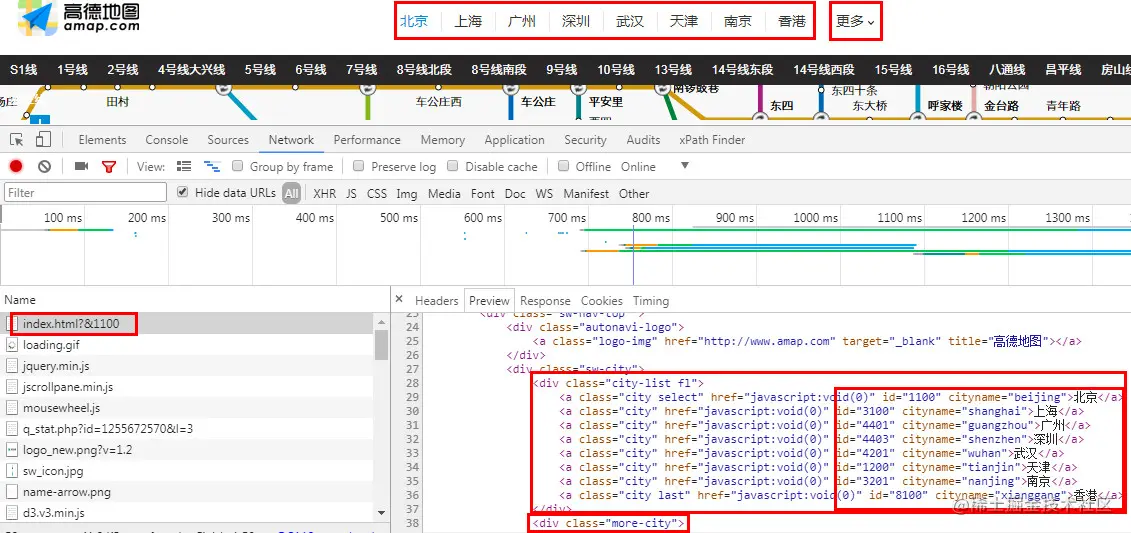The above mainly obtains the city's 「id」,「cityname」 And 「 name 」.

URL for splicing request , Then get the specific information of subway lines .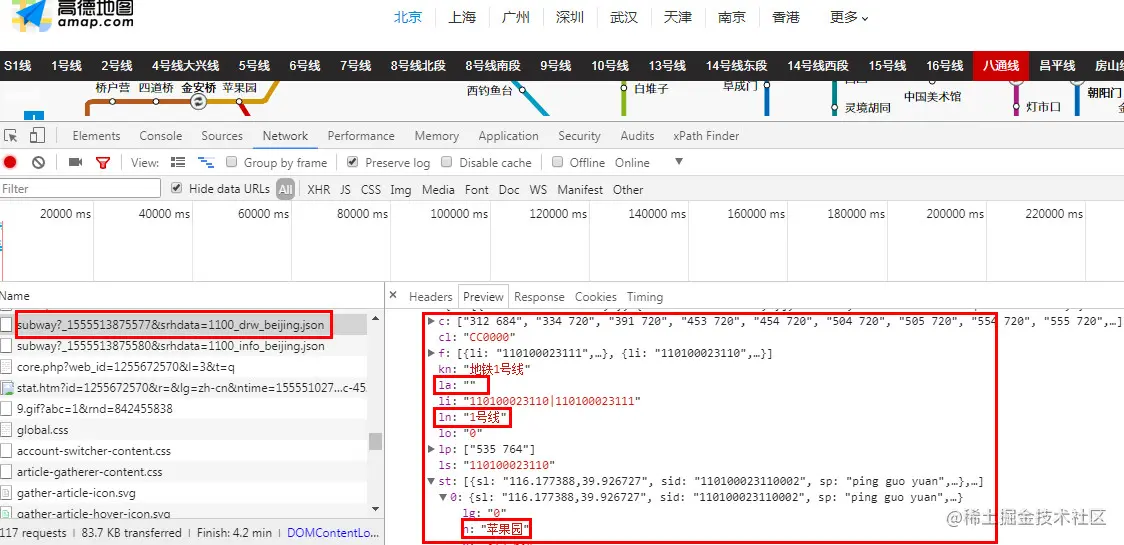Request information found , Get the details of subway lines and stations in each city .

# get data

Specific code

``````import json
import requests
from bs4 import BeautifulSoup

headers = {'user-agent': 'Mozilla/5.0 (Windows NT 6.1; WOW64) AppleWebKit/537.36 (KHTML, like Gecko) Chrome/63.0.3239.132 Safari/537.36'}

def get_message(ID, cityname, name):
"""      Metro line information acquisition      """
url = 'http://map.amap.com/service/subway?_1555502190153&srhdata=' + ID + '_drw_' + cityname + '.json'
html = response.text
for i in result['l']:
for j in i['st']:
#  Judge whether there is a subway line
if len(i['la']) > 0:
print(name, i['ln'] + '(' + i['la'] + ')', j['n'])
with open('subway.csv', 'a+', encoding='gbk') as f:
f.write(name + ',' + i['ln'] + '(' + i['la'] + ')' + ',' + j['n'] + '\n')
else:
print(name, i['ln'], j['n'])
with open('subway.csv', 'a+', encoding='gbk') as f:
f.write(name + ',' + i['ln'] + ',' + j['n'] + '\n')

def get_city():
"""      City information acquisition      """
url = 'http://map.amap.com/subway/index.html?&1100'
html = response.text
#  code
html = html.encode('ISO-8859-1')
html = html.decode('utf-8')
soup = BeautifulSoup(html, 'lxml')
#  City list
res1 = soup.find_all(class_="city-list fl")
res2 = soup.find_all(class_="more-city-list")
for i in res1.find_all('a'):
#  City ID value
ID = i['id']
#  City Pinyin name
cityname = i['cityname']
#  City name
name = i.get_text()
get_message(ID, cityname, name)
for i in res2.find_all('a'):
#  City ID value
ID = i['id']
#  City Pinyin name
cityname = i['cityname']
#  City name
name = i.get_text()
get_message(ID, cityname, name)

if __name__ == '__main__':
get_city()
Copy code ``````

Obtain data and display results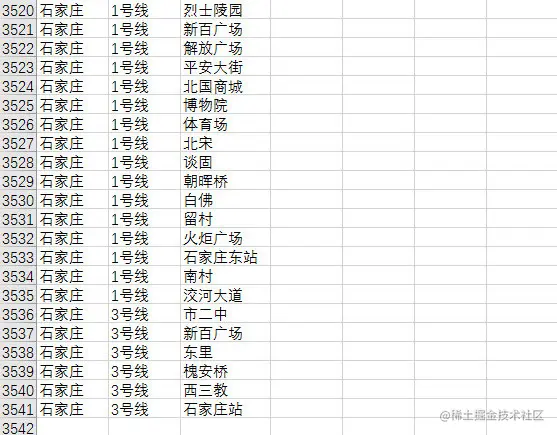3541 A subway station

# Data visualization

Clean the data first , Remove duplicate transfer station information .

``````from wordcloud import WordCloud, ImageColorGenerator
from pyecharts import Line, Bar
import matplotlib.pyplot as plt
import pandas as pd
import numpy as np
import jieba

#  Set column names to align with data
pd.set_option('display.unicode.ambiguous_as_wide', True)
pd.set_option('display.unicode.east_asian_width', True)
#  Show 10 That's ok
pd.set_option('display.max_rows', 10)
#  Subway lines in various cities
df_line = df.groupby(['city', 'line']).count().reset_index()
print(df_line)
Copy code ``````

Grouping by city and subway lines , Get the total number of subway lines in the country .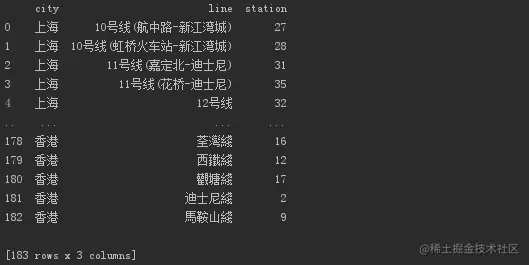183 A subway line

``````def create_map(df):
#  Make a map
value = [i for i in df['line']]
attr = [i for i in df['city']]
geo = Geo(" Distribution of metro cities opened ", title_pos='center', title_top='0', width=800, height=400, title_color="#fff", background_color="#404a59", )
geo.add("", attr, value, is_visualmap=True, visual_range=[0, 25], visual_text_color="#fff", symbol_size=15)
geo.render(" Distribution of metro cities opened .html")

def create_line(df):
"""  Generate the number and distribution of urban subway lines  """
title_len = df['line']
bins = [0, 5, 10, 15, 20, 25]
level = ['0-5', '5-10', '10-15', '15-20', '20 above ']
len_stage = pd.cut(title_len, bins=bins, labels=level).value_counts().sort_index()
#  Generate a histogram
attr = len_stage.index
v1 = len_stage.values
bar = Bar(" Number and distribution of subway lines in each city ", title_pos='center', title_top='18', width=800, height=400)
bar.render(" Number and distribution of subway lines in each city .html")

#  Number of subway lines in each city
df_city = df_line.groupby(['city']).count().reset_index().sort_values(by='line', ascending=False)
print(df_city)
create_map(df_city)
create_line(df_city)
Copy code ``````

City data of Metro has been opened , And the number of subway lines in each city .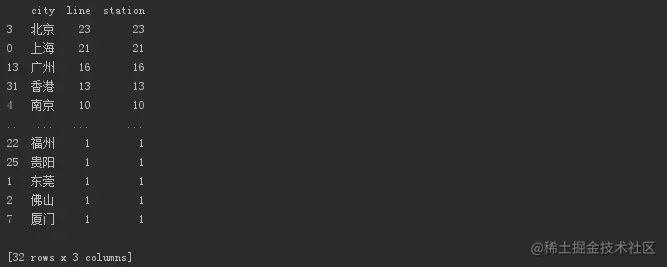32 Cities open subways

Urban distribution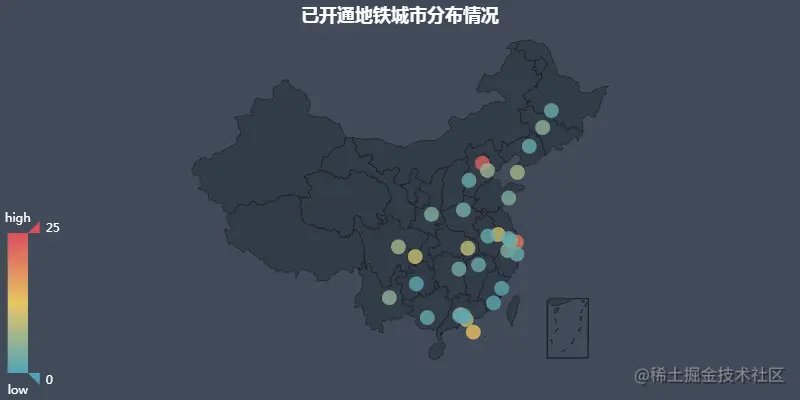Most of them are provincial capitals , There are also some cities with strong economic strength .

Number and distribution of lines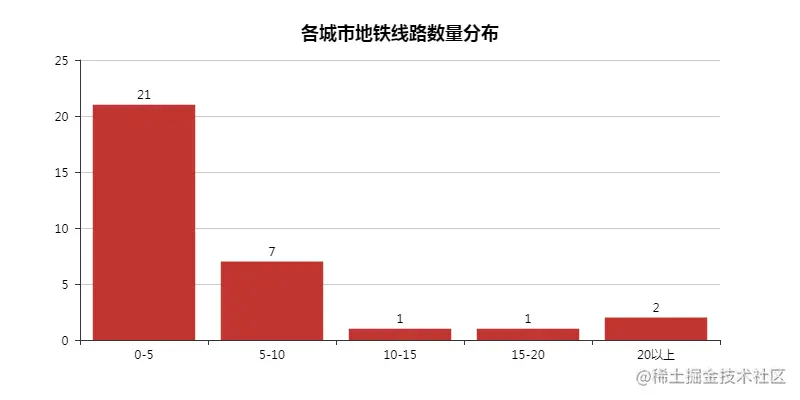You can see that most of them are still 「0-5」 At this stage , Of course, at least 1 line .

``````#  Which city has the most subway stations on which line
print(df_line.sort_values(by='station', ascending=False))
Copy code ``````

Which city has the most subway stations on which line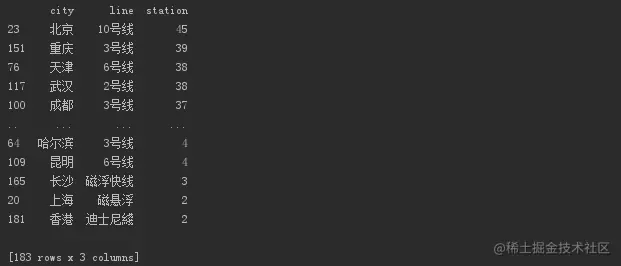Beijing 10 Line 1 , Chongqing 3 Line 2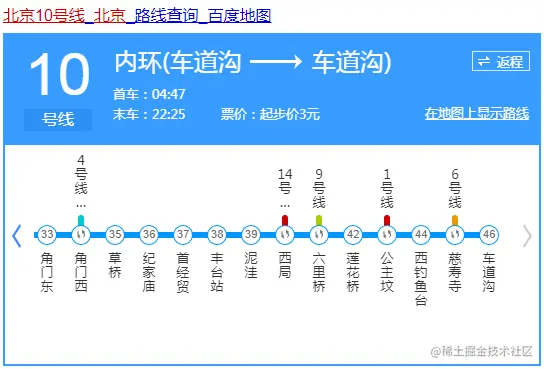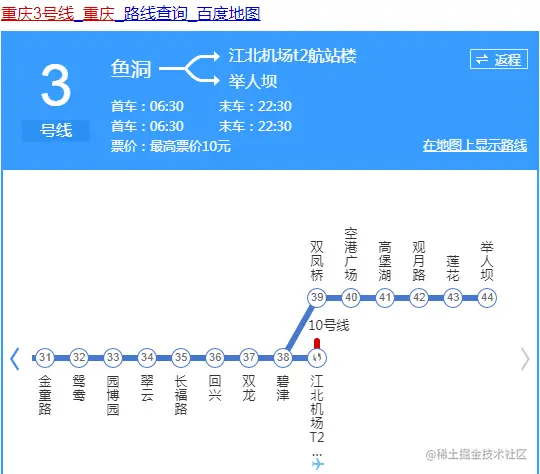Remove duplicate transfer station data

``````#  Remove the subway data of repeated transfer stations
df_station = df.groupby(['city', 'station']).count().reset_index()
print(df_station)
Copy code ``````

contain 3034 A subway station

Reduce the proximity 400 A subway station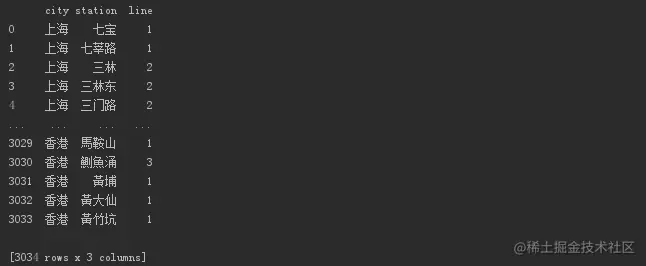Next, let's see which city has the most subway stations

``````#  Count the number of subway stations in each city ( Duplicate transfer stations have been removed )
print(df_station.groupby(['city']).count().reset_index().sort_values(by='station', ascending=False))
Copy code ``````

There are so many subway stations in Wuhan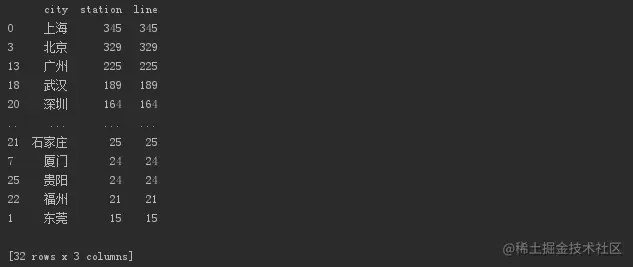Realize the operation in the new weekly , Generate Metro noun cloud

``````def create_wordcloud(df):
"""  Generate Metro noun cloud  """
#  participle
text = ''
for line in df['station']:
text += ' '.join(jieba.cut(line, cut_all=False))
text += ' '
wc = WordCloud(
background_color='white',
font_path='C:\Windows\Fonts\ Huakangli golden black W8.TTF',
max_words=1000,
max_font_size=150,
min_font_size=15,
prefer_horizontal=1,
random_state=50,
)
wc.generate_from_text(text)
img_colors = ImageColorGenerator(backgroud_Image)
wc.recolor(color_func=img_colors)
#  What are the high frequency words
process_word = WordCloud.process_text(wc, text)
sort = sorted(process_word.items(), key=lambda e: e, reverse=True)
print(sort[:50])
plt.imshow(wc)
plt.axis('off')
wc.to_file(" Subway noun cloud .jpg")
print(' Word cloud generated successfully !')

create_wordcloud(df_station)
Copy code ``````

Cloud map of exhibition words